Select Page

# Solutions for MCQ CBSE 12 Science Maths Determinant in Hindi

Solutions for MCQ CBSE 12 Science Maths Determinant in Hindi to enable students to get Solutions in a narrative video format for the specific question.

Expert Teacher provides Solutions for MCQ CBSE 12 Science Maths Determinant through Video Solutions in Hindi language. This video solution will be useful for students to understand how to write an answer in exam in order to score more marks. This teacher uses a narrative style for a question from Determinant not only to explain the proper method of answering question, but deriving right answer too.

Please find the question below and view the Solution in a narrative video format.

Question:

Solution Video in Hindi:

You can select video Solutions from other languages also. Please check Solutions in ( English )

## Similar Questions from CBSE, 12th Science, Maths, Determinant

Question 2 : Evaluate the determinant:. (View Answer Video)

Question 3 :  Using the properties of determinants, evaluate. (View Answer Video)

Question 4 : Evaluate the determinant:. (View Answer Video)

Question 5 :  Find the values of x, if. (View Answer Video)

### Continuity and Differentiability

Question 1 : Differentiate the functionwith respect to x. (View Answer Video)

Question 2 : Differentiate the functionwith respect to x. (View Answer Video)

Question 3 : If, findin terms of y alone. (View Answer Video)

Question 4 :  Find the second order derivative of the function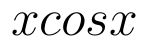. (View Answer Video)

Question 5 : If x and y are connected parametrically by the equation, without eliminating the parameter, find. (View Answer Video)

### Vector Algebra

Question 1 : Find a vector in the direction ofthat has magnitude 7 units.   (View Answer Video)

Question 2 : Find the value of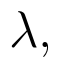if the points with position vectorsandare coplanar.  (View Answer Video)

Question 3 : Find a vector in the direction of vectorwhich has magnitude 21 units.  (View Answer Video)

Question 4 : Write the position vector of the point which divides the join of points with position vectorsandin the ratio 2:1.  (View Answer Video)

Question 5 :  Write a vector in the direction of the vectorthat has magnitude 9 units.   (View Answer Video)

### Probability

Question 1 : If P(A)=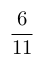, P(B)=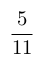and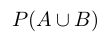=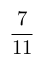, find. (View Answer Video)

Question 2 : If P(A)=0.8, P(B)=0.5 and=0.4, find. (View Answer Video)

Question 3 : Compute P(A/B) if P(B) =0.5 and P(A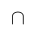B)=0.32. (View Answer Video)

Question 4 : If E and F are two events such that,, Find P(not E and not F). (View Answer Video)